Thursday , July 7 2022# NCERT 5th Class (CBSE) Mathematics: Fractions

(b) 2/3×1/7 = 2/21

1/7×2/3 = 2/21

(c) 3/4×7/15 = 21/60 = 7/20
or
3/4×7/15

Step 1. Find a common factor between any numerator and the denominator in the given question: 3/4×7/15 (3 and 15 have the common factor 3)

Step 2. Divide that numerator and the denominator by the common factor and write the quotient.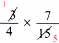Step 3. Multiply the new numerators and denominators.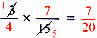Step 4. Write in lowest terms if not already in lowest term = 7/20

(d) 9/25×10/18 = ?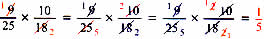9/25×10/18 = 1/5

### C. Multiplying More Than Two Fractions

When you multiply more than two fractions, you can multiply any two fractions first and then multiply the product with the remaining fractions.

3/4×5/6×4/15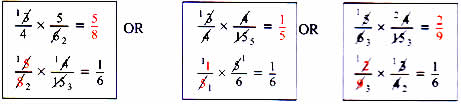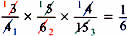## चुनौती हिमालय की 5th NCERT CBSE Hindi Rimjhim Ch 18

चुनौती हिमालय की 5th Class NCERT CBSE Hindi Book Rimjhim Chapter 18 प्रश्न: लद्दाख जम्मू-कश्मीर राज्य में …

### One comment

1.I need worksheet for Class 5th Mathematics.